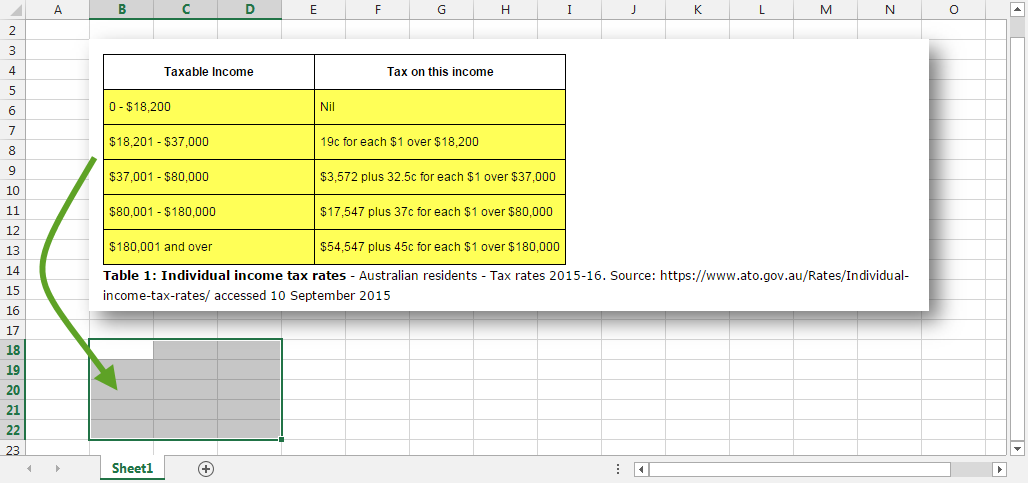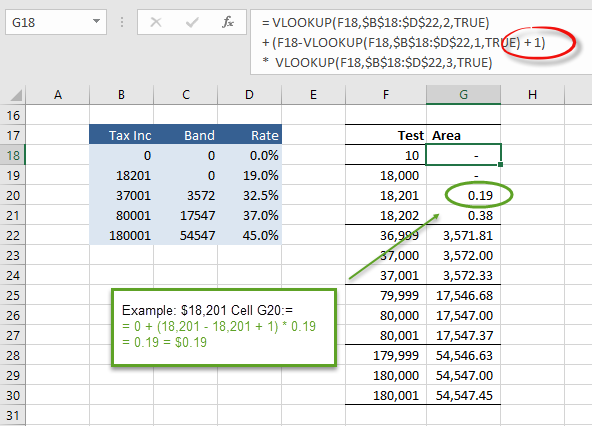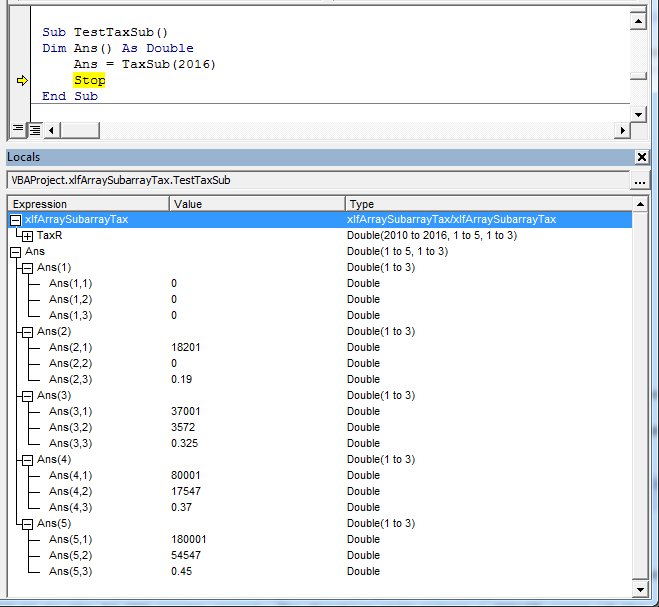# xlf QandA series

## Arrays, and sub-arrays, with application to tax rates

### The QUESTION

Using the VBE:

1. Tax rates array: Write a Private procedure to assign a set to tax rates to a VBA array. The rates apply to an Australian resident individual, and are for tax years 2010 to 2016 inclusive (excluding the Medicare and other levies). The array is static, with dimensions (2010 to 2016, 1 to 5, 1 to 3). In the first dimension, 2010 refers to tax rates for the tax year 2009-10, and so on. Use assignment statements to populate the array

2. Sub array: Write a Private function procedure to return the second and third dimension (ie. a two dimensional sub array) for a specific tax year, from the array developed in part a. Assume that this function is only called from VBA, and not from a worksheet

3. Tax: Write a Public function named Tax, categorized in the WS Financial group, that returns the tax values, based on an amount of taxable income, and a date within a specific tax year. For example, Tax(54000, DATEVALUE("31-Dec-2010")) will apply the Tax Schedule for 2010-11 to the 54,000 income amount. The function will return a #VALUE error if the date is out of range

## Preliminary

A set of tax rates are required. These are available from the Australian Taxation Office (ATO) web site and are included here for your reference.

Source: Australian Taxation Office

As a preliminary exercise it is suggested that the tax rates for one year are entered into a 5 row by 3 column array in a worksheet. The Excel range should be suitable for use with the Excel VLOOKUP function. As an example, the 2015-16 tax rates (this is the first dimension, 2016 index developed later in VBA), is shown in the yellow section of Table 1 in figure 1. The table "information" needs its appropriate numeric values to be entered into the 15 available cells in the area shown by the selection on the worksheet "Sheet1". You can then use VLOOKUP (in Excel) to return the amount of tax payable. Once you understand the logic, you can then assign the tax values to the elements of the 3-D array in VBA.

Code structure: the public Tax function in part c, calls the 2-D array from the private function in part b, which in turn uses the 3-D array from part a.Fig 1: Tax 2015-16 - table and Excel array (testing area). Source: xlf-if...then...else moduleFig 2: Tax 2015-16 - as a worksheet 5 x 3 array (B18:D22 with column B in ascending order). In the case of an approximate match, the VLOOKUP function will look for the next largest value that is less than the lookup value.

Note: set the category lower band at 18201, 37001, ... to clearly indicate that the 18,201st dollar is taxable at 19%, and the 37,001st dollar is taxable at 32.5%, ...

QUESTION a Tax rates: Write a Private procedure to assign a set to tax rates to a VBA array. The rates apply to an Australian resident individual, and are for tax years 2010 to 2016 inclusive (excluding the Medicare levy). The array is static, with dimensions (2010 to 2016, 1 to 5, 1 to 3). In the first dimension, 2010 refers to tax rates for the tax year 2009-10, and so on. Use assignment statements to populate the array.

1. Assign a set of tax rates to a 7 x 5 x 3 array in VBA. A 3-D array can be thought of as comprising rows x columns x depth
2. Make the array values available to the sub array extraction procedure in code 2

Code 1: Part (a) - Module level declaration, and TaxRates sub procedure
Option Explicit
Dim TaxR(2010 To 2016, 1 To 5, 1 To 3) As Double

Private Sub TaxRates()
'' 2015 to 2016
TaxR(2016, 1, 1) = 0
TaxR(2016, 1, 2) = 0
TaxR(2016, 1, 3) = 0

TaxR(2016, 2, 1) = 18201
TaxR(2016, 2, 2) = 0
TaxR(2016, 2, 3) = 0.19

TaxR(2016, 3, 1) = 37001
TaxR(2016, 3, 2) = 3572
TaxR(2016, 3, 3) = 0.325

TaxR(2016, 4, 1) = 80001
TaxR(2016, 4, 2) = 17547
TaxR(2016, 4, 3) = 0.37

TaxR(2016, 5, 1) = 180001
TaxR(2016, 5, 2) = 54547
TaxR(2016, 5, 3) = 0.45

'' 2014 to 2015
TaxR(2015, 1, 1) = 0
TaxR(2015, 1, 2) = 0
TaxR(2015, 1, 3) = 0

TaxR(2015, 2, 1) = 18201
TaxR(2015, 2, 2) = 0
TaxR(2015, 2, 3) = 0.19

TaxR(2015, 3, 1) = 37001
TaxR(2015, 3, 2) = 3572
TaxR(2015, 3, 3) = 0.325

TaxR(2015, 4, 1) = 80001
TaxR(2015, 4, 2) = 17547
TaxR(2015, 4, 3) = 0.37

TaxR(2015, 5, 1) = 180001
TaxR(2015, 5, 2) = 54547
TaxR(2015, 5, 3) = 0.45

'' 2013 to 2014
TaxR(2014, 1, 1) = 0
TaxR(2014, 1, 2) = 0
TaxR(2014, 1, 3) = 0

TaxR(2014, 2, 1) = 18201
TaxR(2014, 2, 2) = 0
TaxR(2014, 2, 3) = 0.19

TaxR(2014, 3, 1) = 37001
TaxR(2014, 3, 2) = 3572
TaxR(2014, 3, 3) = 0.325

TaxR(2014, 4, 1) = 80001
TaxR(2014, 4, 2) = 17547
TaxR(2014, 4, 3) = 0.37

TaxR(2014, 5, 1) = 180001
TaxR(2014, 5, 2) = 54547
TaxR(2014, 5, 3) = 0.45

'' 2012 to 2013
TaxR(2013, 1, 1) = 0
TaxR(2013, 1, 2) = 0
TaxR(2013, 1, 3) = 0

TaxR(2013, 2, 1) = 18201
TaxR(2013, 2, 2) = 0
TaxR(2013, 2, 3) = 0.19

TaxR(2013, 3, 1) = 37001
TaxR(2013, 3, 2) = 3572
TaxR(2013, 3, 3) = 0.325

TaxR(2013, 4, 1) = 80001
TaxR(2013, 4, 2) = 17547
TaxR(2013, 4, 3) = 0.37

TaxR(2013, 5, 1) = 180001
TaxR(2013, 5, 2) = 54547
TaxR(2013, 5, 3) = 0.45

'' 2011 to 2012
TaxR(2012, 1, 1) = 0
TaxR(2012, 1, 2) = 0
TaxR(2012, 1, 3) = 0

TaxR(2012, 2, 1) = 6001
TaxR(2012, 2, 2) = 0
TaxR(2012, 2, 3) = 0.15

TaxR(2012, 3, 1) = 37001
TaxR(2012, 3, 2) = 4650
TaxR(2012, 3, 3) = 0.3

TaxR(2012, 4, 1) = 80001
TaxR(2012, 4, 2) = 17550
TaxR(2012, 4, 3) = 0.37

TaxR(2012, 5, 1) = 180001
TaxR(2012, 5, 2) = 54550
TaxR(2012, 5, 3) = 0.45

'' 2010 to 2011
TaxR(2011, 1, 1) = 0
TaxR(2011, 1, 2) = 0
TaxR(2011, 1, 3) = 0

TaxR(2011, 2, 1) = 6001
TaxR(2011, 2, 2) = 0
TaxR(2011, 2, 3) = 0.15

TaxR(2011, 3, 1) = 37001
TaxR(2011, 3, 2) = 4650
TaxR(2011, 3, 3) = 0.3

TaxR(2011, 4, 1) = 80001
TaxR(2011, 4, 2) = 17550
TaxR(2011, 4, 3) = 0.37

TaxR(2011, 5, 1) = 180001
TaxR(2011, 5, 2) = 54550
TaxR(2011, 5, 3) = 0.45

'' 2009 to 2010
TaxR(2010, 1, 1) = 0
TaxR(2010, 1, 2) = 0
TaxR(2010, 1, 3) = 0

TaxR(2010, 2, 1) = 6001
TaxR(2010, 2, 2) = 0
TaxR(2010, 2, 3) = 0.15

TaxR(2010, 3, 1) = 37001
TaxR(2010, 3, 2) = 4650
TaxR(2010, 3, 3) = 0.3

TaxR(2010, 4, 1) = 80001
TaxR(2010, 4, 2) = 17580
TaxR(2010, 4, 3) = 0.38

TaxR(2010, 5, 1) = 180001
TaxR(2010, 5, 2) = 55850
TaxR(2010, 5, 3) = 0.45

End Sub



• Line 2: Declare an array named TaxR at the Module level (Declarations area). The array is static, this means its dimensions are specified in the declaration. First dimension index (2010 to 2016), second dimension index (1 to 5), and third dimension index (1 to 3). This array is initialized with default values when any procedure in the Module is run. Once populated by the TaxRates procedure, the values are retained during the current session and thus available to code 2
• Line 4: Private Sub - does not appear in the Macro list in Excel. Can be tested by setting a Break Point (or inserting a Stop statement), run by pressing F5, then viewing the array in the Locals window
• Lines 6 to 8: Assign values to the first column, depth 1, 2, and 3
• Lines 10 to 12: Assign values to the second column, depth 1, 2, and 3. Then continue the assignment process ...

QUESTION b Sub array: Write a Private function procedure to return the second and third dimension (ie. a two dimensional sub array) for a specific tax year, from the array developed in part a. Assume that this function is only called from VBA, and not from a worksheet.

1. Create a function procedure with one argument, the tax year as a 4 digit integer, of type Integer
2. The function returns a 5 x 3 array. Use type Variant to accommodate a multi value return item
3. The sub array will be dynamic to provide flexibility, however, a static array is equally acceptable as it is 5 rows x 3 columns in all cases provided here

Code 2: Part (b) - TaxSub function procedure. One argument TaxYear as type Integer
Private Function TaxSub(TaxYear As Integer) As Variant
Dim NoRows As Integer
Dim NoCols As Integer
Dim i As Integer, j As Integer
Dim TaxSubArr() As Double

If TaxR(2016, 5, 3) = 0 Then TaxRates

NoRows = UBound(TaxR, 2)
NoCols = UBound(TaxR, 3)
ReDim TaxSubArr(1 To NoRows, 1 To NoCols)

For i = 1 To NoRows
For j = 1 To NoCols
TaxSubArr(i, j) = TaxR(TaxYear, i, j)
Next j
Next i

TaxSub = TaxSubArr

End Function


• Line 160: Procedure declaration. Name: TaxSub. One argument TaxYear, and return type Variant to accommodate an array
• Lines 161 to 163: Variable declarations
• Line164: Declare an dynamic array named TaxSubArr. The array is dynamic because the dimension(s) are left blank
• Line166: Check if the module level array TaxR has zero elements. If yes then call the TaxRates procedure (code 1) to assign the tax rates to the 3-D array
• Lines 168: Get the index number of the upper element in the second dimension of the array named TaxR and assign to the NoRows variable. Returns 5
• Lines 169: Get the index number of the upper element in the third dimension of the array named TaxR and assign to the NoCols variable. Returns 3
• • UBound (Upper Bound) - Syntax: UBound(arrayname [,dimension])
• Lines 170: Now that the dimensions are retrieved, the values can be used to Redim the dynamic array TaxSubArr
• Lines 172 to 176: A double For...Next loop - process elements in rows and columns
• Line 174: Right hand side - for a particular year specified by the TaxYear argument, return values from the second and third dimension of the 3-D array. Assign each element to the first and second dimensions of the 2-D named TaxSubArr
• Line178: Assign the TaxSubArr array values to the function return value

Code 3: Part (b) - TextTaxSub sub procedure. A test procedure to call the TaxSub function
Sub TestTaxSub()
Dim Ans() As Double
Ans = TaxSub(2016)
Stop
End Sub


• Line 186: Declare a dynamic array of type Double. In this instance the array is not Redim'ed because it will inherit the dimensions of the assigned array in line 187
• Line 187: Right hand side - call the TaxSub function with TaxYear argument set to 2016. TaxSub returns a Variant array, that is assigned to Ans array
• Line 188: The Stop statement causes code execution to halt. Yellow background and arrow in gutter. Use the Locals window to view the array (figure 2)
•Fig 2: Locals window showing Ans array with elements expanded

QUESTION c Tax: Tax: Write a Public function named Tax, categorized in the WS Financial group, that returns the tax values, based on an amount of taxable income, and a date within a specific tax year. For example, Tax(54000, DATEVALUE("31-Dec-2010")) will apply the Tax Schedule for 2010-11 to the 54,000 income amount. The function will return a #VALUE error if the date is out of range.

• Three suggested solutions are provided - other combinations are also possible
• The procedures for the 3-D array and the sub array are used as a data source for the tax rates

• This part of the example incorporates two logical processes:
1. Convert the date to a tax year pointer for the appropriate tax array
2. Construction a code segment to return the tax payable from that particular tax table

• Code 4: 1. Select...Case with individual tax years, and 2. the Excel VLOOKUP function with a 2-D array
• Code 5: 1. Simplified Select...Case with VBA Year, and Month function, and 2. the Excel VLOOKUP function with a 2-D array
• Code 6: 1. Simplified If...Then...ElseIf with VBA Year, and Month function, and 2. If...Then...ElseIf and 2-D array elements

Code 4: Part (c) - Tax function procedure (version 1)
Public Function Tax(Income As Long, Date_ As Date) As Variant
Dim i As Long
Dim Year As Integer
Dim T() As Double

On Error GoTo ErrHandler

Select Case Date_
Case DateValue("1 July 2015") To DateValue("30 June 2016")
Year = 2016
Case DateValue("1 July 2014") To DateValue("30 June 2015")
Year = 2015
Case DateValue("1 July 2013") To DateValue("30 June 2014")
Year = 2014
Case DateValue("1 July 2012") To DateValue("30 June 2013")
Year = 2013
Case DateValue("1 July 2011") To DateValue("30 June 2012")
Year = 2012
Case DateValue("1 July 2010") To DateValue("30 June 2011")
Year = 2011
Case DateValue("1 July 2009") To DateValue("30 June 2010")
Year = 2010
End Select

T = TaxSub(Year)
i = Income

With Application
Tax = .Vlookup(i, T, 2) + (i - (.Vlookup(i, T, 1) + 1)) * .Vlookup(i, T, 3)
End With

Exit Function
ErrHandler:
Tax = CVErr(xlErrValue)
End Function


• Lines 212 to 227: tax year pointer Select...Case with start and end dates for tax year. 16 code lines
• Line 229: call the TaxSub function with argument Year (a 4 digit Integer) from the tax year pointer segment
• Line 233: tax table lookup using Excel's VLOOKUP function. 1 code line

Code 5: Part (c) - Tax function procedure (version 2)
Public Function Tax2_(Income As Long, Date_ As Date) As Variant
Dim i As Long
Dim Mth As Integer, Yr As Integer
Dim T() As Double

On Error GoTo ErrHandler

Mth = Month(Date_)
Select Case Mth
Case 1 To 6: Yr = Year(Date_)
Case 7 To 12: Yr = Year(Date_) + 1
End Select

T = TaxSub(Yr)
i = Income

With Application
Tax2_ = .Vlookup(i, T, 2) + (i - (.Vlookup(i, T, 1) + 1)) * .Vlookup(i, T, 3)
End With

Exit Function
ErrHandler:
Tax2_ = CVErr(xlErrValue)
End Function


• Lines 252 to 256: tax year pointer 5 code lines
• Lines 254 and 255: call the TaxSub function with argument Year (a 4 digit Integer) from the tax year pointer segment
• Line 262: tax table lookup using Excel's VLOOKUP function. The same statement as code 4

Code 6: Part (c) - Tax function procedure (version 3)
Public Function Tax3_(Income As Long, Date_ As Date) As Variant
Dim i As Long
Dim Mth As Integer
Dim Yr As Integer
Dim T() As Double

On Error GoTo ErrHandler

Mth = Month(Date_)
If Mth >= 1 Or Mth <= 6 Then
Yr = Year(Date_)
ElseIf Mth >= 7 Or Mth <= 12 Then
Yr = Year(Date_) + 1
End If

T = TaxSub(Yr)
i = Income

If i < T(2, 1) Then
Tax3_ = T(1, 2) + (i - (T(1, 1) + 1)) * T(1, 3)
ElseIf i < T(3, 1) Then
Tax3_ = T(2, 2) + (i - (T(2, 1) + 1)) * T(2, 3)
ElseIf i < T(4, 1) Then
Tax3_ = T(3, 2) + (i - (T(3, 1) + 1)) * T(3, 3)
ElseIf i < T(5, 1) Then
Tax3_ = T(4, 2) + (i - (T(4, 1) + 1)) * T(4, 3)
Else
Tax3_ = T(5, 2) + (i - (T(5, 1) + 1)) * T(5, 3)
End If

Exit Function
ErrHandler:
Tax3_ = CVErr(xlErrValue)
End Function


• Lines 278 to 283: tax year pointer 5 code lines
• Line 285: call the TaxSub function with argument Year (a 4 digit Integer) from the tax year pointer segment
• Line 288 to 298: tax table values returned by direct reference to elements

Code 7: Part (c) - TestTax sub procedure
Sub TestTax()
Dim Ans As Double, Ans2_ As Double, Ans3_ As Double

Ans = Tax(180001, DateValue("21 December 2009"))
Ans2_ = Tax2_(180001, DateValue("21 December 2009"))
Ans3_ = Tax3_(180001, DateValue("21 December 2009"))
Stop

End Sub


• Lines 313 to 315: returns values for each of Tax, Tax2_, and Tax3_. View the values in the Locals window

Code 8: - categorized in the worksheet Financial group
Private Sub AddTaxToFinancialCategory()
Dim FuncName As String
Dim FuncDesc As String
Dim FuncCategory As String
'' Dim FuncArgDesc(1 To 2) As String

On Error GoTo ErrHandler

FuncName = "Tax"
FuncDesc = "Returns amount of tax payable"
FuncCategory = 1 ' Financial category
'' FuncArgDesc(1) = "the amount of taxable income"
'' FuncArgDesc(2) = "the serial date value in the tax year"

Application.MacroOptions _
Macro:=FuncName, _
Description:=FuncDesc, _
Category:=FuncCategory
'' ArgumentDescriptions:=FuncArgDesc

Exit Sub
ErrHandler:
End Sub